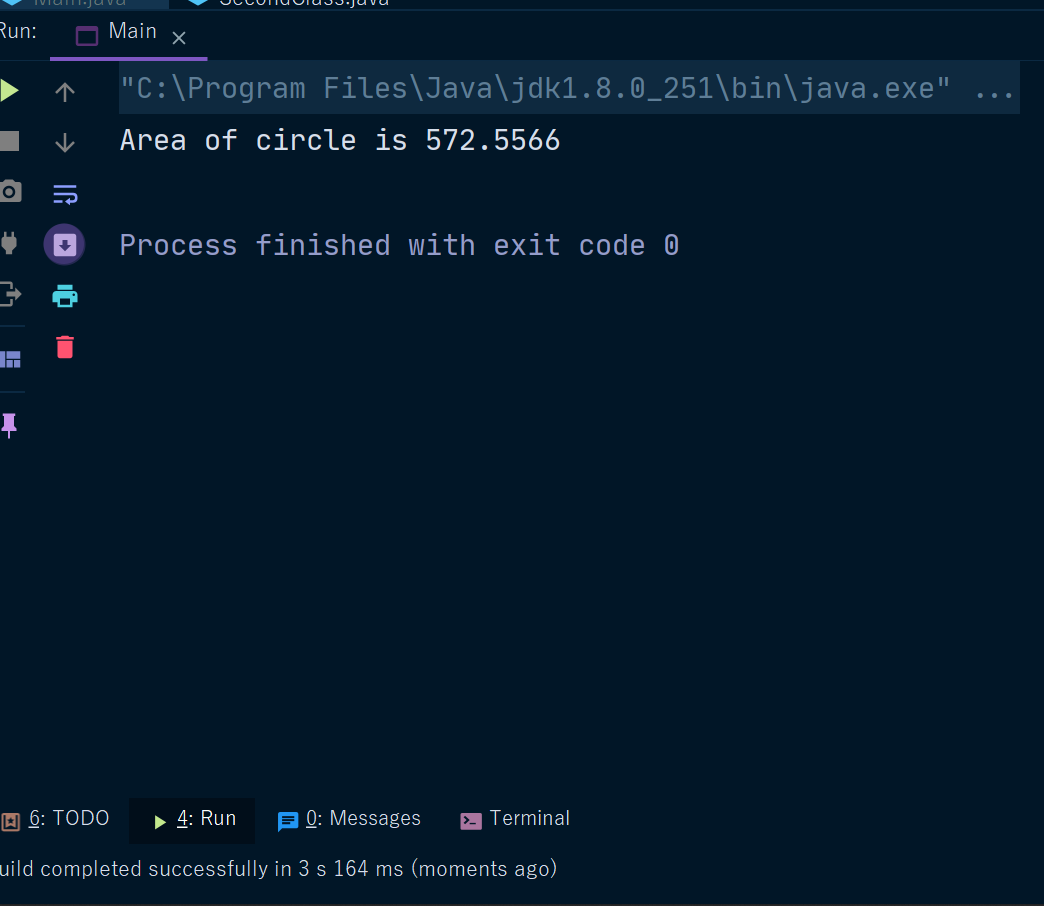Java Double Type

# Java Double Type

Double Type In Java

Double is a keyword used to declare double data type variables by putting double keywords before the identifier or the variable name.

The double data type can store fractional numbers from 1.7e−308 to 1.7e+308.
We use floating points when the space required is 8 bytes or less.
Double precision, as denoted by the double keyword, uses 64 bits to store a value.
Double precision is actually faster than single precision on some modern processors that have been optimized for high-speed mathematical calculations.

All transcendental math functions, such as sin( ), cos( ), and sqrt( ), return double values. When you need to maintain accuracy over many iterative calculations or are manipulating large-valued numbers, double is the best choice.

Now we will see Examples -

Here is a short program that uses double variables to compute the area of a circle:

Example 1 -

 duble num =30.20d; System.out.println(num);

Example -

 // Compute the area of a circle. class Area { public static void main(String args[]) { double pi, r, a; r = 13.5; // radius of circle pi = 3.1416; // pi, approximately a = pi * r * r; // compute area System.out.println("Area of circle is " + a); } }

Output -